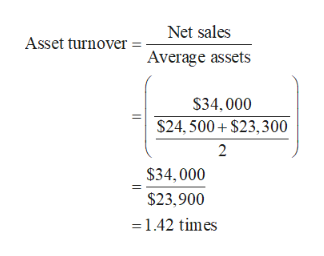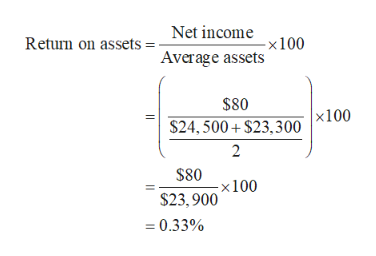# Suppose during 2022 that Federal Express reported the following information (in millions): net sales of \$34,000 and net income of \$80. Its balance sheet also showed total assets at the beginning of the year of \$24,500 and total assets at the end of the year of \$23,300.Calculate the asset turnover and return on assets. (Round asset turnover to 2 decimal places, e.g. 6.25 and return on assets to 1 decimal place, e.g. 17.5%.)Asset turnover enter the asset turnover rounded to 2 decimal places timesReturn on assets enter the return on assets in percentages rounded to 2 decimal places%

Question
78 views

Suppose during 2022 that Federal Express reported the following information (in millions): net sales of \$34,000 and net income of \$80. Its balance sheet also showed total assets at the beginning of the year of \$24,500 and total assets at the end of the year of \$23,300.

Calculate the asset turnover and return on assets. (Round asset turnover to 2 decimal places, e.g. 6.25 and return on assets to 1 decimal place, e.g. 17.5%.)

 Asset turnover enter the asset turnover rounded to 2 decimal places times Return on assets enter the return on assets in percentages rounded to 2 decimal places %
check_circle

Step 1

Calculate the asset turnover.help_outlineImage TranscriptioncloseNet sales Asset turnover Average assets \$34,000 \$24, 500 + \$23,300 2 \$34,000 \$23,900 =1.42 times fullscreen
Step 2

Calculate the return on as...help_outlineImage TranscriptioncloseNet income Return on assets : -×100 Average assets \$80 |x100 \$24, 500+ \$23,300 2 \$80 -x100 \$23,900 = 0.33% fullscreen

### Want to see the full answer?

See Solution

#### Want to see this answer and more?

Solutions are written by subject experts who are available 24/7. Questions are typically answered within 1 hour.*

See Solution
*Response times may vary by subject and question.
Tagged in